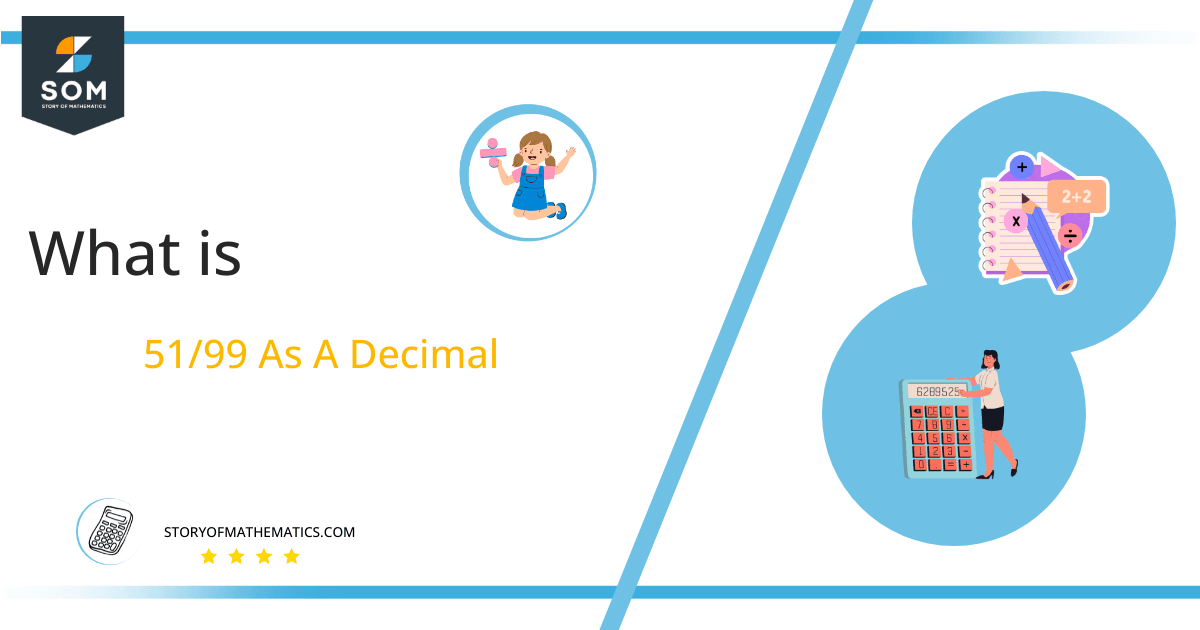# What Is 51/99 as a Decimal + Solution With Free Steps

The fraction 51/99 as a decimal is equal to 0.515.

A rational number that contains a pattern of digits that repeat infinitely after the decimal point is called a repeating decimal. It exists when decimal numbers repeat forever. The fraction 51/99 is a repeating decimal fraction.Here, we are more interested in the division types that result in a Decimal value, as this can be expressed as a Fraction. We see fractions as a way of showing two numbers having the operation of Division between them that result in a value that lies between two Integers.

Now, we introduce the method used to solve said fraction to decimal conversion, called Long Division, which we will discuss in detail moving forward. So, let’s go through the Solution of fraction 51/99.

## Solution

First, we convert the fraction components, i.e., the numerator and the denominator, and transform them into the division constituents, i.e., the Dividend and the Divisor, respectively.

This can be done as follows:

Dividend = 51

Divisor = 99

Now, we introduce the most important quantity in our division process: the Quotient. The value represents the Solution to our division and can be expressed as having the following relationship with the Division constituents:

Quotient = Dividend $\div$ Divisor = 51 $\div$ 99

This is when we go through the Long Division solution to our problem. The following figure shows the solution for fraction 51/99.Figure 1

## 51/99 Long Division Method

We start solving a problem using the Long Division Method by first taking apart the division’s components and comparing them. As we have 51 and 99, we can see how 51 is Smaller than 99, and to solve this division, we require that 51 be Bigger than 99.

This is done by multiplying the dividend by 10 and checking whether it is bigger than the divisor or not. If so, we calculate the Multiple of the divisor closest to the dividend and subtract it from the Dividend. This produces the Remainder, which we then use as the dividend later.

Now, we begin solving for our dividend 51, which after getting multiplied by 10 becomes 510.

We take this 510 and divide it by 99; this can be done as follows:

510 $\div$ 99 $\approx$ 5

Where:

99 x 5 = 495

This will lead to the generation of a Remainder equal to 510 – 495 = 15. Now this means we have to repeat the process by Converting the 15 into 150 and solving for that:

150 $\div$ 99 $\approx$ 1

Where:

99 x 1 = 99

This, therefore, produces another Remainder which is equal to 150 – 99 = 51. Now this means we have to repeat the process by Converting the 51 into 510 and solving for that:

510 $\div$ 99 $\approx$ 5

Where:

99 x 5 = 495

Finally, we have a Quotient generated after combining the three pieces of it as 0.515, with a Remainder equal to 15.Images/mathematical drawings are created with GeoGebra.